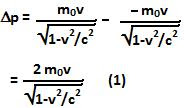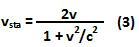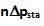## Thursday, January 7, 2010

### Irodov Problem 1.377In the frame of reference of the sphere

As seen from the frame of reference of the sphere, the sphere itself is stationary. The gas particles are however moving at a speed v towards it. Let us now consider the collision of the sphere with a single particle. As asked in the question consider a section of the sphere that is normal to the direction of velocity. Assuming that the sphere is extremely large compared to the gas particles, in case of an elastic collision, the gas particle will simply bounce off with the same velocity v in the opposite direction. The net change in momentum of the gas particle is given by,As seen from the reference frame of the particles there are n particles per unit volume. However, the same unit volume with n particles as seen by an observer moving with the sphere is shrunk by a factor ofsince space itself would have shrunk by this factor in the direction of motion. In other words, the density of the gas a seen from the observer on the sphere would beSince n' particles change their momentum each second, the net force exerted on the sphere as a reaction will beSolution in reference frame of the particles
As seen from the view of the gas particles, they were initially stationary and the sphere was moving at a velocity v. Since as seen from the sphere's reference frame, the final velocity (velocity after collision) was 2v, their final velocity as seen by a stationary observer can be obtained by using the relativistic transformation as,This means that the change in momentum from the point of view of a stationary observer is given by,Since the number of particles colliding with the sphere per unit time is n, the net force exerted on the sphere is given by, which evaluates exactly the same as calculated from the point of view of an observer on the sphere!